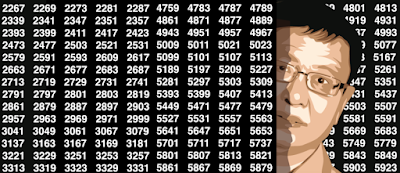## Friday, June 5, 2020

### Bernouilli Lattice Models - Connection to Poisson Processes

Bernouilli lattice processes may be one of the simplest examples of point processes, and can be used as an introduction to learn about more complex spatial processes that rely on advanced measure theory for their definition. In this article, we show the differences and analogies between Bernouilli lattice processes on the standard rectangular or hexagonal grid, and the Poisson process, including convergence of discrete lattice processes to continuous Poisson process, mainly in two dimensions. We also illustrate that even though these lattice processes are purely random, they don't look random when seen with the naked eye.
We discuss basic properties such as the distribution of the number of points in any given area, or the distribution of the distance to the nearest neighbor. Bernouilli lattice processes have been used as models in financial problems. Most of the papers on this topic are hard to read, but here we discuss the concepts in simple English. Interesting number theory problems about sums of squares, deeply related to these lattice processes, are also discussed. Finally, we show how to identify if a particular realization is from a Bernouilli lattice process, a Poisson process, or a combination of both.
See below a realization of a Bernouilli process on the regular hexagonal lattice. The main feature of such a process is that the point locations are fixed, not random. But whether a point is "fired" or not (that is, marked in blue) is purely random and independent of whether any other point is fired or not. The probability for a point to be fired is a Bernouilly variable of parameter p
Figure 1: realization of Bernouilli hexagonal lattice process
More sophisticated models, known as Markov random fields, allows for neighboring points to be correlated. They are useful in image analysis.
To the contrary, Poisson processes assume that the point locations are random. The points being fired are uniformly distributed on the plane, and not restricted to integer or grid coordinates. In short, Bernouilli lattice processes are discrete approximations to Poisson processes. Below is an example of a realization of a Poisson process.
Math / data science articles by the same author:
Here is a selection of articles pertaining to experimental math and data science:

### Machine Learning Perspective on the Twin Prime Conjecture

This article focuses on the machine learning aspects of the problem, and the use of pattern recognition techniques leading to interesting,...